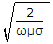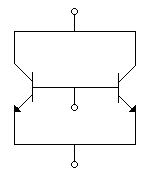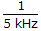Courses

# Test: Electronic Devices And Circuits - 3

## 25 Questions MCQ Test Electronic Devices | Test: Electronic Devices And Circuits - 3

Description
This mock test of Test: Electronic Devices And Circuits - 3 for Electrical Engineering (EE) helps you for every Electrical Engineering (EE) entrance exam. This contains 25 Multiple Choice Questions for Electrical Engineering (EE) Test: Electronic Devices And Circuits - 3 (mcq) to study with solutions a complete question bank. The solved questions answers in this Test: Electronic Devices And Circuits - 3 quiz give you a good mix of easy questions and tough questions. Electrical Engineering (EE) students definitely take this Test: Electronic Devices And Circuits - 3 exercise for a better result in the exam. You can find other Test: Electronic Devices And Circuits - 3 extra questions, long questions & short questions for Electrical Engineering (EE) on EduRev as well by searching above.
QUESTION: 1

### Which of these has 3 layers?

Solution:

p layer, i layer and n layer.

QUESTION: 2

### As per Einstein's equation, the velocity of emitted electron in photoelectric emission is given by the equation

Solution:

Some electrons have less energy than Fermi level E and, therefore, more energy than Uw to escape.

Therefore, this equation has inequality sign.

QUESTION: 3

### For an P-channel enhancement type MOSFET determine the drain current if K = 0.278 x 10-3A/V2, VGS = -4V, VT = -2V, Voltage equivalent at 27�C = 26 mV.

Solution:

Drain current Id  = k (|Vgs| - |VT|)2

= 0.278 x 10-3(4 - 2)2

= 1.11 mA.

QUESTION: 4

The skin depth of copper is found to be 66 mm at 1 MHz at a certain temperature. At the same temperature and at 2 MHz, the skin depth would be approximately

Solution:

Skin depth =QUESTION: 5

When p-n junction is reverse biased the holes in p material move towards the junction and electrons in n material move away from the junction.

Solution:

Both holes and electrons move away from junction.

QUESTION: 6

A semiconductor diode is biased in forward direction and carrying current I. The current due to holes in p material is

Solution:

Small current due to minority carriers also. Therefore hole current is less than.

QUESTION: 7

Between which regions does BJT act like switch?

Solution:

In cut off mode a BJT is an open switch and in saturation mode it is a closed switch.

QUESTION: 8

Assertion (A): When a photoconductive device is exposed to light, its bulk resistance increases.

Reason (R): When exposed to light, electron hole pairs are generated in the photoconductive device.

Solution:

A is wrong because exposure to light decreases bulk resistance.

QUESTION: 9

Which of the following elements act as donor impurities?

1. Gold
2. Phosphorus
3. Boron
4. Antimony
5. Arsenic
6. Indium
Select the answer using the following codes :
Solution:

Only antimony, arsenic and phosphorous are pentavalent.

QUESTION: 10

Light dependent resistor is

Solution:

Resistance of LDR depends on light.

QUESTION: 11

The breakdown voltage in a zener diode

Solution:

Therefore, it is used in voltage regulator circuits.

QUESTION: 12

A varactor diode is used for

Solution:

Its voltage dependent capacitance is used for tuning.

QUESTION: 13

One eV = 1.602 x 10-19 joules.

Solution:

One electron has a charge 1.602 x 10-19 C, when it falls through 1 V, energy is 1 eV = 1.602 x 10-19 J.

QUESTION: 14

Assertion (A): When VDS is more than rated value the drain current in a JFET is very high.

Reason (R): When VDS is more than rated value, avalanche breakdown occurs.

Solution:

Avalanche breakdown causes high current.

QUESTION: 15

If ib is plate current, eb is plate voltage and ec is grid voltage the v-i curve of a vacuum triode is
ib = 0.003 (eb + kec)n. Typical values of k and n are

Solution:

k has to be much higher than 1 so that grid is effective in controlling plate current n is not 1 because it is a nonlinear device.

QUESTION: 16

In which material do conduction and valence bands overlap

Solution:

This is the reason for high conductivity of conductors.

QUESTION: 17

For a photoconductor with equal electron and hole mobilities and perfect ohmic contacts at the ends, an increase in illumination results in

Solution:

Increase in illumination reduces resistance of a photoconductor.

QUESTION: 18

Discrete transistors T1 and T2 having maximum collector current rating of 0.75 amp are connected in parallel as shown in the figure, this combination is treated as a single transistor to carry a total current of 1 ampere, when biased with self bias circuit. When the circuit is switched on, T1 draws 0.55 amps and T2 draws 0.45 amps. If the supply is kept on continuously, ultimately it is very likely thatSolution:

T1 draws 0.55 A and T2 draws 0.45 A, T1 will get more heated and correct increase. Ultimately, I1 = 1A and I2 = 0.

QUESTION: 19

The number of p-n junctions in a semiconductor diode are

Solution:

Semiconductor diode has one p-n junction, BJT has two and SCR has three p-n junctions.

QUESTION: 20

Assertion (A): A high junction temperature may destroy a diode.

Reason (R): As temperature increases the reverse saturation current increases.

Solution:

Both A and R are correct. The diode is destroyed due to high reverse current.

QUESTION: 21

When a diode is not conducting, its bias is

Solution:

Diode conducts only when forward biased. There is no conduction when bias is zero or reverse.

QUESTION: 22

The SCR would be turned OFF by voltage reversal of applied anode-cathode ac supply of frequency of

Solution:

For inverter grade SCR, typical turn off time (toff) is 5 to 50 μsec while 50 to 100 μsec for converter grade SCR.

Hence supply frequency of 5 kHz will turn off the SCR by voltage reversal
because= 200 μsec.

QUESTION: 23

The number of valence electrons in a donor atom is

Solution:

Therefore, it can donate one electron.

QUESTION: 24

An electron rises through a voltage of 100 V. The energy acquired by it will be

Solution:

When an electron rises through 1 V, energy = 1 eV.

QUESTION: 25

Measurement of hall coefficient enables the determination of

Solution:

Hall effect can be used to find type and concentration of charge carriers.